The output (x) of logic circuit shown in figure will be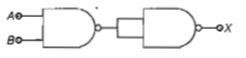(a) $X=\stackrel{=}{A}·\stackrel{=}{B}$

(b) $X=\overline{A.B}$

(c)$X=A·B$

(d)$X=\overline{A+B}$

Concept Questions :-

Logic gates
High Yielding Test Series + Question Bank - NEET 2020

Difficulty Level:

Two ideal diodes are connected to a battery as shown in the circuit. The current supplied by the battery is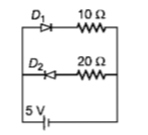(a)0.75A                              (b)zero

(c)0.25A                               (d)0.5A

Concept Questions :-

PN junction
High Yielding Test Series + Question Bank - NEET 2020

Difficulty Level:

In a CE transistor amplifier, the audio signal voltage across the collector resistance of 2  If the base resistance is 1 $k\Omega$ and the current amplification of the transistor is 100, the input signal voltage is

1. 0.1 V                                         2. 1.0 V

3. 1mV                                         4. 10mV

Concept Questions :-

Transistor
High Yielding Test Series + Question Bank - NEET 2020

Difficulty Level:

C and Si both have same lattice structure,having 4 bonding electrons in each.However, C is insulator whereas Si is intrinsic semiconductor. This is because

1. in case of C, the valence band is not completely filled at absolute zero temperature

2. in case of C,the condition band is partly filled even at absolute zero temperature

3. the four bonding electrons in the case of C lie in the second orbit,Whereas in the case of Si they lie in the third

4 .the four bonding electrons in the case of C lie in the third orbit, whereas for Si they lie in the fourth orbit

Concept Questions :-

Types of semiconductors
High Yielding Test Series + Question Bank - NEET 2020

Difficulty Level:

Transfer characteristic [output voltage $\left({V}_{0}\right)$ vs input voltage $\left({V}_{i}\right)$] for a base biased transistor in CE configuration is as shown in the figure.For using transistor as a switch, it is used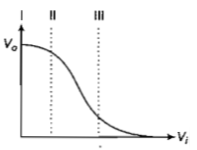(a) in region III

(b) both in region (I) and (III)

(c) in region II

(d) in region I

Concept Questions :-

Transistor
High Yielding Test Series + Question Bank - NEET 2020

Difficulty Level:

The figure shows a logic circuit with two inputs A and B and the output C.The voltage wave forms across A, B and C are as given.The logic circuit gate is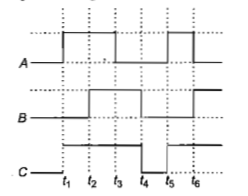1. OR gate                                          2. NOR gate

3. AND gate                                        4. NAND gate

Concept Questions :-

Logic gates
High Yielding Test Series + Question Bank - NEET 2020

Difficulty Level:

The input resistance of a silicon transistor is

100 $\mathrm{\Omega }$. Base current is changed by 40 $\mathrm{\mu A}$

which results in a change in collector current

by 2 mA. This transistor is used as a common-

emitter amplifier with a load resistance of 4 k$\mathrm{\Omega }$.

The voltage gain of the amplifier is

1. 2000

2. 3000

3. 4000

4. 1000

Concept Questions :-

Transistor
High Yielding Test Series + Question Bank - NEET 2020

Difficulty Level:

To get an output Y=1 in given circuit, which

of the following input will be correct ?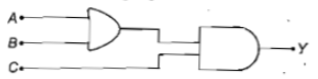A     B      C                   A     B     C

1.  1     0       0             2.  1     0     1

3. 1      1       0             4.  0     1     0

Concept Questions :-

Logic gates
High Yielding Test Series + Question Bank - NEET 2020

Difficulty Level:

Symbolic representation of four logic gates

are shown as

(i)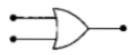(ii)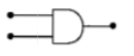(iii)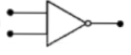(iv)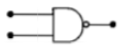Pick out which ones are for AND, NAND and

NOT gates, respectively.

(a) (iii), (ii)and (i)

(b) (iii), (ii) and (iv)

(c) (ii), (iv) and (iii)

(d) (ii), (iii) and (iv)

Concept Questions :-

Logic gates
High Yielding Test Series + Question Bank - NEET 2020

Difficulty Level:

If a small amount of antimony is added to germanium crystal

(a) the antimony becomes an acceptor atom

(b) there will be more free electrons than holes in the semiconductor

(c) its resistance is increased

(d) it becomes a p-type semiconductor

Concept Questions :-

Types of semiconductors